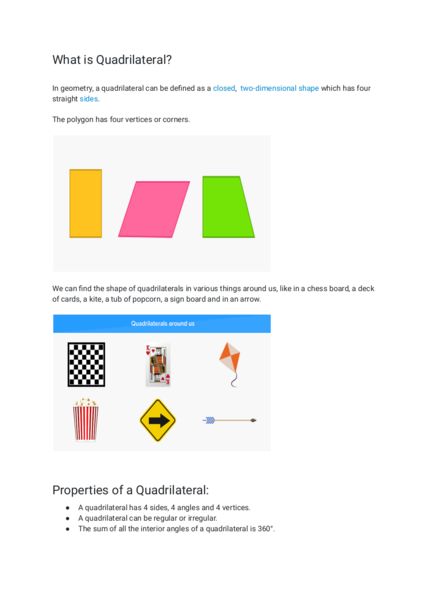Contributed by:this pdf covers types of quadrilaterals and their property. n geometry a quadrilateral is a four-sided polygon, having four edges and four corners. The word is derived from the Latin words Quadri, a variant of four, and latus, meaning "side".
In geometry, a quadrilateral can be defined as a closed, two-dimensional shape which has four
straight sides.
The polygon has four vertices or corners.
We can find the shape of quadrilaterals in various things around us, like in a chess board, a deck
of cards, a kite, a tub of popcorn, a sign board and in an arrow.
● A quadrilateral has 4 sides, 4 angles and 4 vertices.
● A quadrilateral can be regular or irregular.
● The sum of all the interior angles of a quadrilateral is 360°.
Quadrilaterals can be classified into Parallelograms, Squares, Rectangles and Rhombuses.
Square, Rectangle and Rhombus are also Parallelograms.
Here’s a list of the types of quadrilaterals with their name, pictures and properties:
Parallelogram Opposite sides are parallel.
Opposites sides are equal.
Opposite angles are equal.
3. Square All sides are equal.
All angles are equal and
measure 90°.
Rectangle Opposite sides are parallel.
Opposites sides are equal.
All angles are equal and
measure 90°.
Rhombus All sides are equal.
Opposite angles are equal.
4. Trapezoid Opposite sides are parallel.